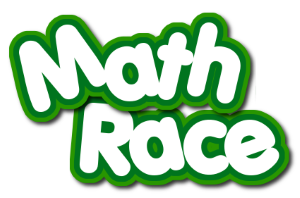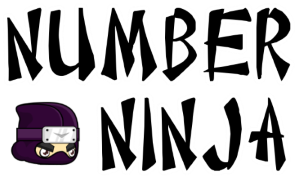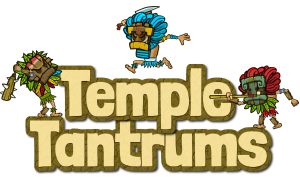## Games sorted by Math Skills

 Addends(Missing numbers in addition and subtraction.)Area & PerimeterBasic Math Facts(Number recognition, addition, subtraction, multiplication, division.)Basic Math Facts(Number recognition, addition, subtraction, multiplication, division.)Basic Math Facts(Missing numbers in addition, subtraction, multiplication, division, and two-step math.)Basic Math Facts(Addition, subtraction, multiplication, division)Basic Math Facts(Addition, subtraction, multiplication, division)Basic Math Facts & Integers(Addition, subtraction, multiplication, division)Basic Math Facts(Addition, subtraction, multiplication, division)Basic Math Facts(Addition, subtraction, multiplication, division)Basic Math Facts(Addition, subtraction, multiplication, division)Basic Math Facts(Number recognition, addition, subtraction, multiplication, division.)Basic Math Facts(Number recognition, addition, subtraction, multiplication, division.)Basic Math Facts(Addition, subtraction, multiplication, division)Basic Math Facts(Addition, subtraction, multiplication, division)Basic Math Facts(Addition, subtraction, multiplication, division)Basic Math Facts(Addition, subtraction, multiplication, division)Comparing Numbers(Greater than or less than)Counting to 10Counting to 10Counting to 5, 10, 15, or 20DecimalsFact FamiliesFactors & Multiples(Prime, odd, & even numbers)FractionsFractionsFractionsFractionsFractionsFractions(Reducing)Inequalities(Factors, multiples, evens & odds)Integers(Positive & Negative Numbers)Money(Counting Coins)Money(Counting Coins)MultiplesNumber SenseNumber OrderSkip CountingNumber PatternsSkip CountingOrder of OperationsAverageRangeRemaindersPatterns & LogicPlace ValuePlace ValuePlace ValuePrime Numbers(Odds & Evens, Place Value)Rounding & EstimatingSkip CountingMultiplesSums & DifferencesTime(Reading a clock, elapsed time.)Time(Reading and elapsed time.)### Math Skills